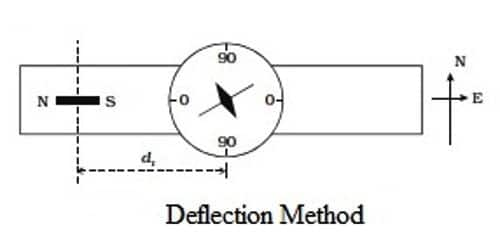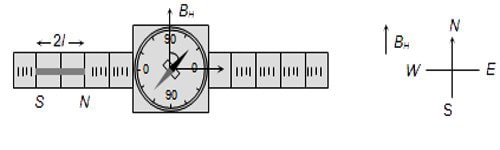# Comparison of Magnetic Moments of Two Bar Magnets in Deflection Method

Magnetic Moments of Two Bar Magnets in Deflection Method:

Deflection magnetometer works on the principle of tangent law. According to tangent law when two uniform magnetic fields act at right angles to each other on a magnetic needle, it comes to rest in the way of resultant field. The deflection magnetometer is placed in Tan A position (Figure). A bar magnet of magnetic moment M1 and length 2Ɩ1 is placed at a distance d1 from the center of the magnetic needle, on one side of the compass box. Since the sensitivity of the magnetometer is more at 45°, the distance of the bar magnet should be chosen such that the deflection lies between 30° and 60°.The readings corresponding to the ends of the aluminum pointer are noted as θ1 and θ2. The magnet is reversed pole to pole and kept at the same distance. Two more readings θ3 and θ4 are noted. By placing the magnet on the other side of the compass box at the same distance, four more readings θ5, θ6, θ7, and θ8 are noted as above. The mean of the eight readings gives a value θI.

The experiment is repeated as above for the second bar magnet of magnetic moment M2 and length 2Ɩ2 by placing at a distance d2. Now the mean of the eight readings gives a value of θII.

Applying tangent law, for the first magnet,

0/4π) * {2M1d1/(d1212)2} = Bh tan θI …. …. …. (1)

and for the second magnet.

0/4π) * {2M2d2/(d1222)2} = Bh tan θII …. …. …. (2)

From the above equations (1) and (2), we get

(M1/M2) = [(d1212)2/(d2222)2] * (d2/d1) * (tan θI/ tan θII)

It is the equation for magnetic moments of two bar magnets in Deflection method.

Deflection magnetometer is an apparatus used to determine the magnetic moment and polar potency of a bar magnet. It is also used to contrast the pole strengths of two bar magnet.

Deflection magnetometer is used to –

• compare magnetic moments
• compare earth’s magnetic inductions
• verify inverse square law and
• determine the horizontal constituent of the earth’s magnetic induction.Fig: Deflection magnetometer apparatus